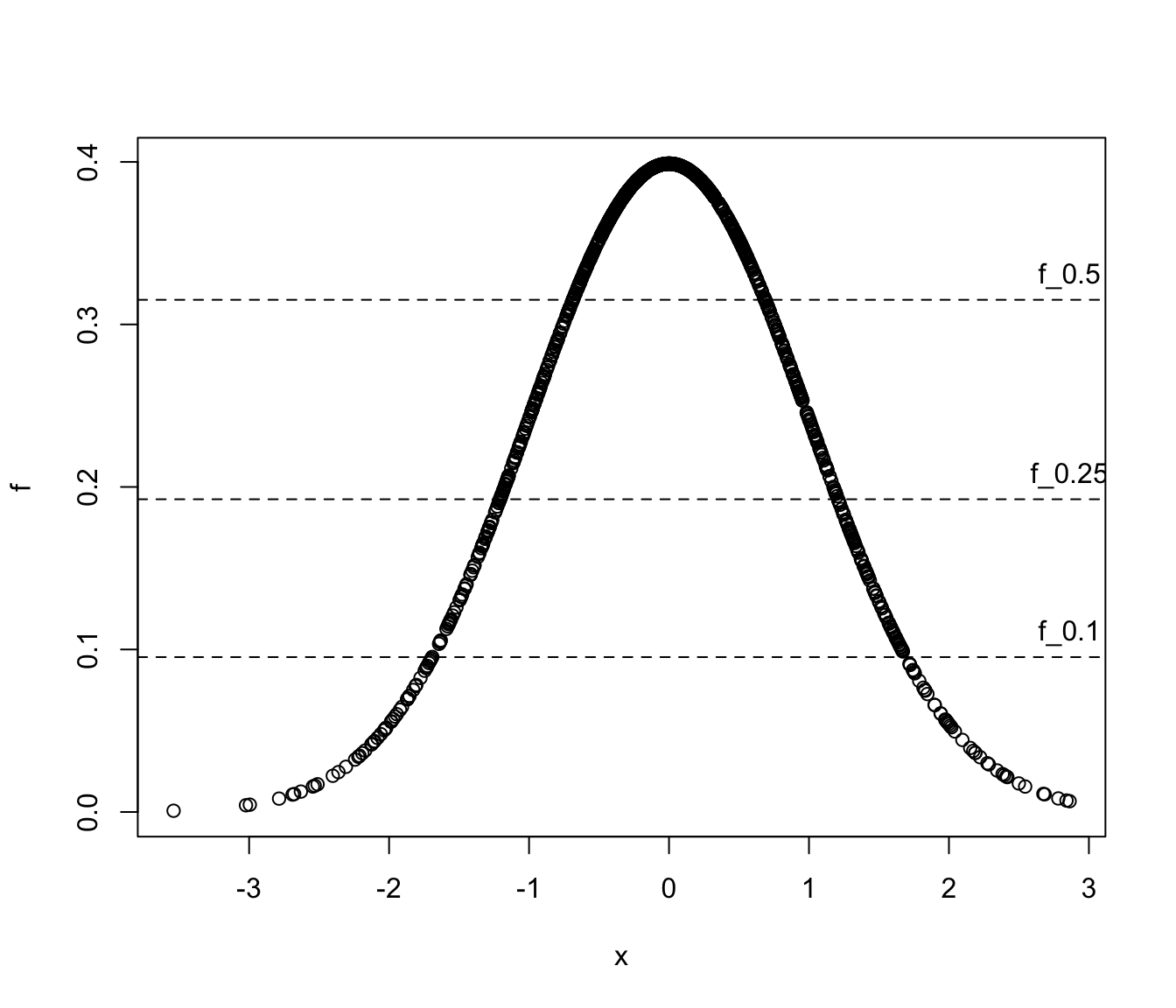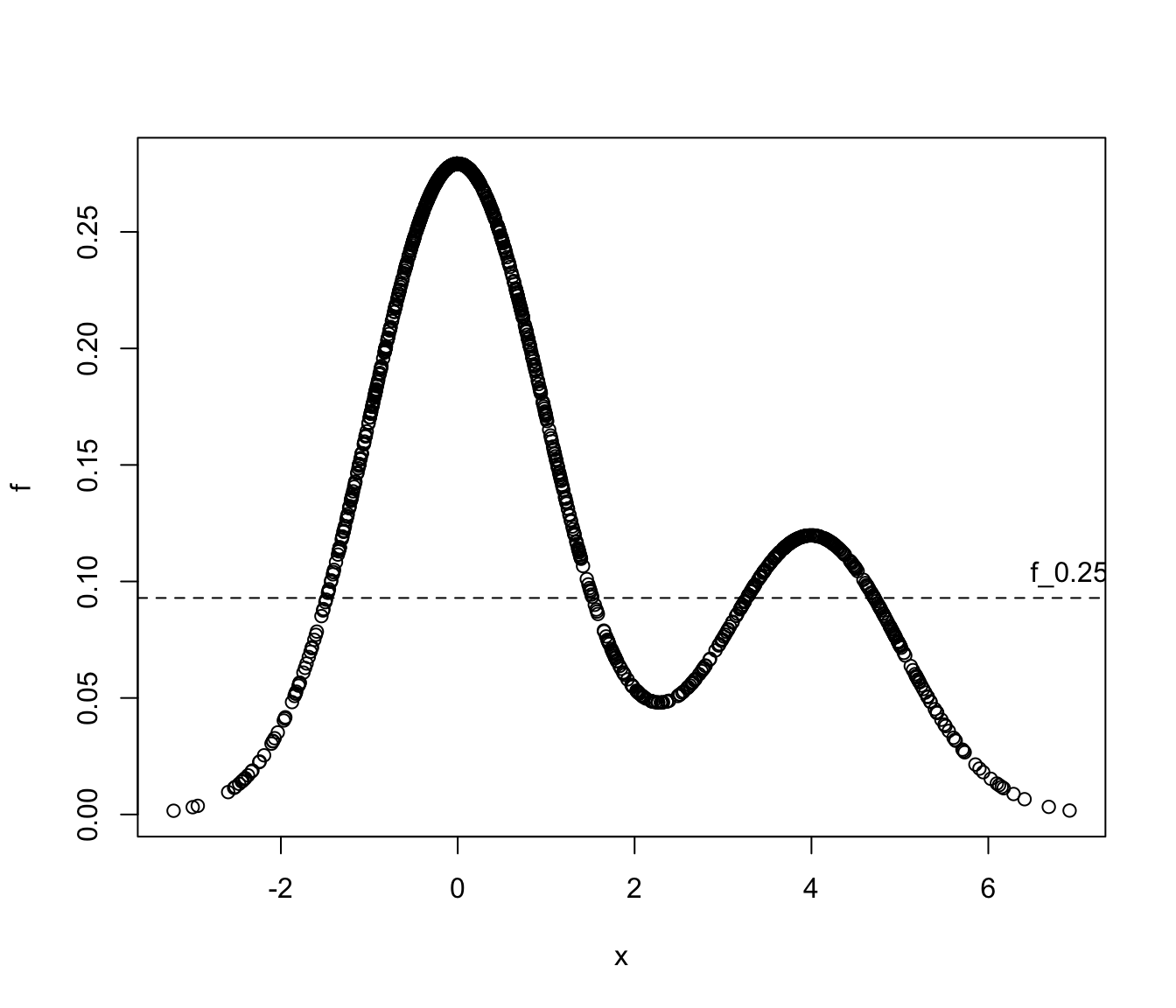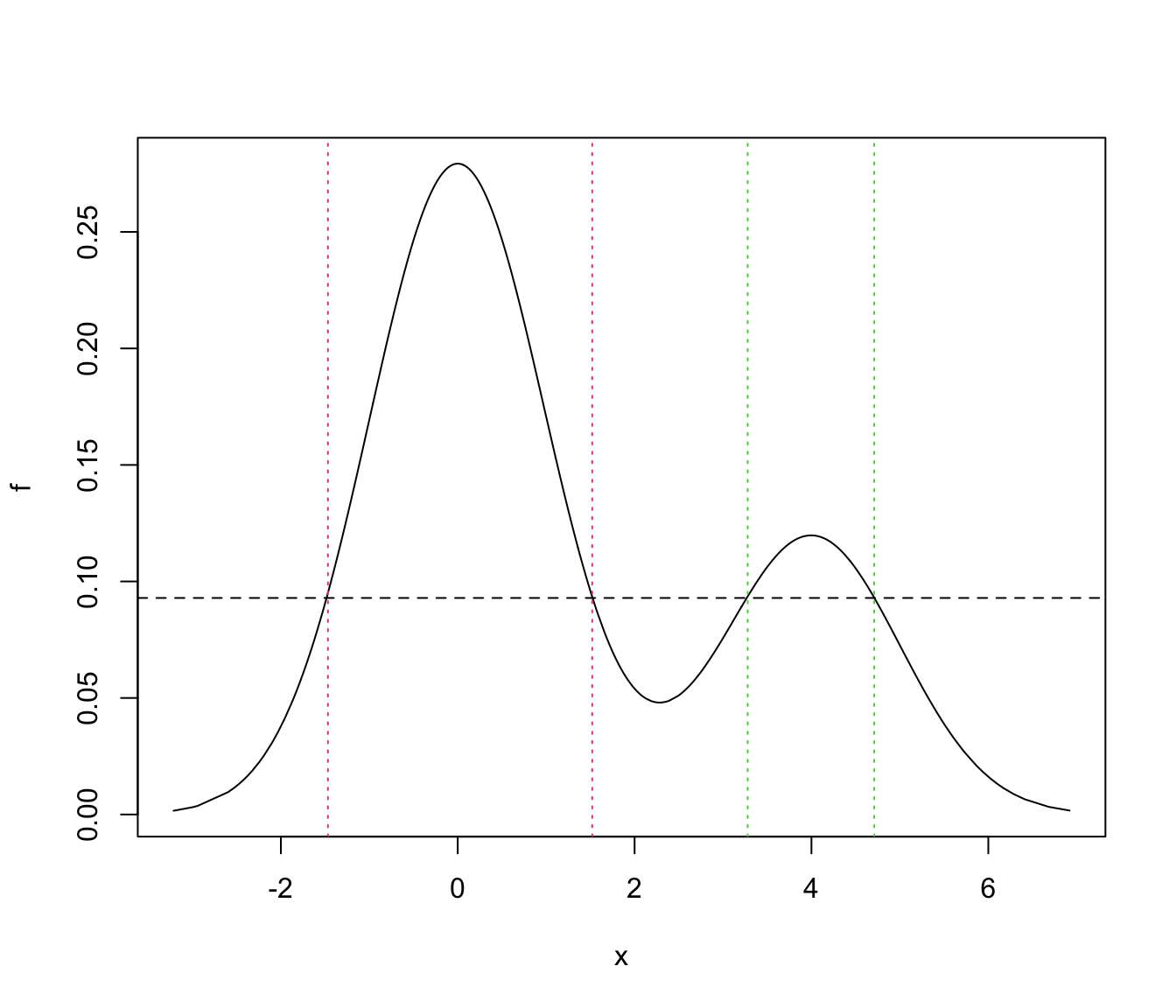Compute the levels of Highest Density Regions (HDRs) for any density and probability levels.

hdrlevels(density, prob)

Arguments

density

A vector of density values computed on a set of (observed) evaluation points.

prob

A vector of probability levels in the range $$[0,1]$$.

Value

The function returns a vector of density values corresponding to HDRs at given probability levels.

Details

From Hyndman (1996), let $$f(x)$$ be the density function of a random variable $$X$$. Then the $$100(1-\alpha)\%$$ HDR is the subset $$R(f_\alpha)$$ of the sample space of $$X$$ such that $$R(f_\alpha) = {x : f(x) \ge f_\alpha }$$ where $$f_\alpha$$ is the largest constant such that $$Pr( X \in R(f_\alpha)) \ge 1-\alpha$$

L. Scrucca

Examples

# Example: univariate Gaussian
x <- rnorm(1000)
f <- dnorm(x)
a <- c(0.5, 0.25, 0.1)
(f_a <- hdrlevels(f, prob = 1-a))
#>       50%       75%       90%
#> 0.3200053 0.2098644 0.1016923

plot(x, f)
abline(h = f_a, lty = 2)
text(max(x), f_a, labels = paste0("f_", a), pos = 3)mean(f > f_a)
#>  0.5
range(x[which(f > f_a)])
#>  -0.6618501  0.6610135
qnorm(1-a/2)
#>  0.6744898

mean(f > f_a)
#>  0.75
range(x[which(f > f_a)])
#>  -1.121584  1.132708
qnorm(1-a/2)
#>  1.150349

mean(f > f_a)
#>  0.9
range(x[which(f > f_a)])
#>  -1.652819  1.650853
qnorm(1-a/2)
#>  1.644854

# Example 2: univariate Gaussian mixture
set.seed(1)
cl <- sample(1:2, size = 1000, prob = c(0.7, 0.3), replace = TRUE)
x <- ifelse(cl == 1,
rnorm(1000, mean = 0, sd = 1),
rnorm(1000, mean = 4, sd = 1))
f <- 0.7*dnorm(x, mean = 0, sd = 1) + 0.3*dnorm(x, mean = 4, sd = 1)

a <- 0.25
(f_a <- hdrlevels(f, prob = 1-a))
#>        75%
#> 0.09291342

plot(x, f)
abline(h = f_a, lty = 2)
text(max(x), f_a, labels = paste0("f_", a), pos = 3)mean(f > f_a)
#>  0.75

# find the regions of HDR
ord <- order(x)
f <- f[ord]
x <- x[ord]
x_a <- x[f > f_a]
j <- which.max(diff(x_a))
region1 <- x_a[c(1,j)]
region2 <- x_a[c(j+1,length(x_a))]
plot(x, f, type = "l")
abline(h = f_a, lty = 2)
abline(v = region1, lty = 3, col = 2)
abline(v = region2, lty = 3, col = 3)